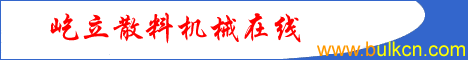The  Slewing  speed adjusting theory of boom type bucket wheel reclaimer              By Liyimin   1988-10                                                               return home  In scope of the slewing angle, the slewing device usually is speed-change type, the method of speed-change is usually that adjust the speed according to the speed-change is in direct proportion with the reciprocal of cosine of angle of rail and speed. We used to use 3-grades speed change. Now, by the advanced frequency conversion motor and DC motor, continuous speed adjusting is widely used in bucket wheel stacker and reclaimer. The depth per layer and moment feeding depth can be calculated by the slewing speed. As showed by figure 1, h means depth/per layer, m0  means the feeding depth when slewing angle is 0, △r means the feeding depth at some slewing angle, V means the linear speed of wheel boom rotate at some angle, a’ means the position of slewing angle, unit is degree. The reclaiming capacity of some angle can be calculated by following formula:         Q=h△rVq/3600t/h     (1) In the formula: Q—reclaiming capacity  t/h; q—material density t/m3;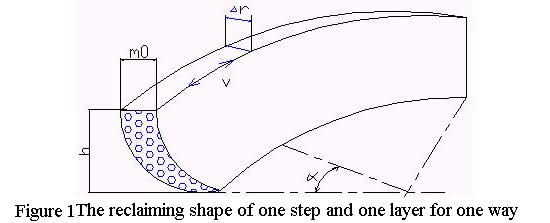The trace of  wheel bucket horizontal digging is shown in figure 2, the cross point of y1 and x in the figure is the slewing center of last slewing, the cross  point of y and x is the slewing center of feeding 0 m, △r is the feeding depth when slewing angle is a’. r is the slewing radius + wheel radius, m: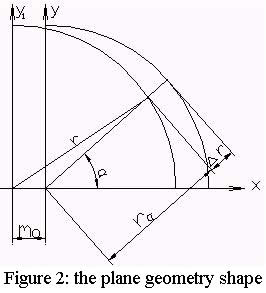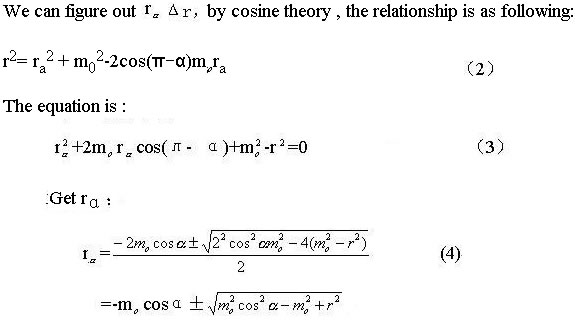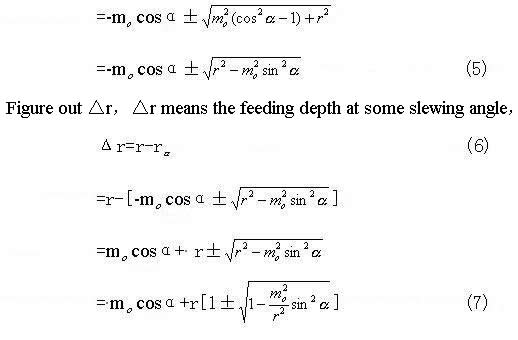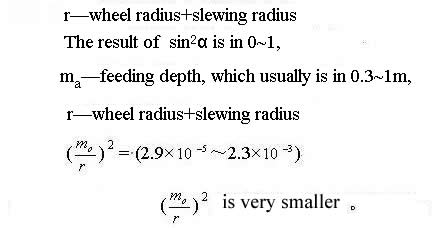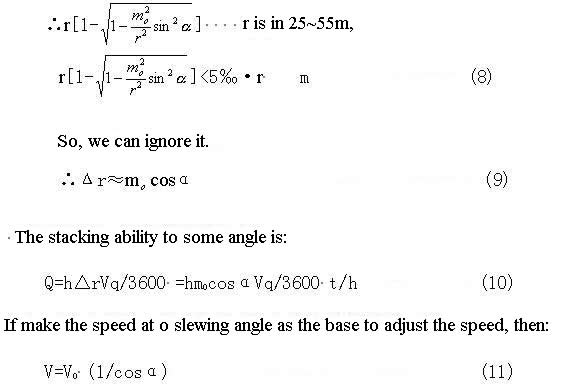则 ：     Q=hm0cosα(1/cosα)q/3600=hm0V0q/3600   t/h             (12)     The reclaiming ability is a stable value, to get a stable reclaiming capacity. In the practice use, due to the 1/cosa’ is infinite great at 90°, the angle of adjust speed is in 0~70°, when the angle is larger than 70°, the speed is same with the speed at 70°to avoid the impact and shake from the over high speed.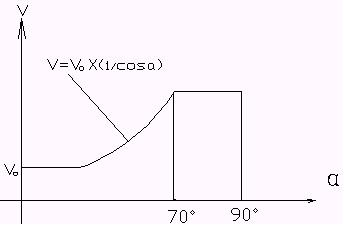Figure 3 , the curve of slewing angle and speed Result:    1, The slewing speed of slewing arm stacker and reclaimer can be adjusted according the variety rule which is in direct proportion with the rule of the reciprocal of cosine. 2, There is a certain degree difference between this speed adjusting method and practical accurate value, but it is enough for engineering, and it can simplify the curve of speed change. 3, The changed slewing speed is in 0-70degreen , the speed in 70-90degreen is fixed. Copyright  ©  by BULK-ONWEB, BULK MACHINERY TECHNIQUE  2001-2005         版权所有：《散料机械技术》《屹立散料机械在线》                                        未经许可授权不可转载和使用本站稿件内容                                  return home